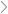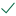Questions & AnswersMicroeconomicsSarah receives utility from days spent traveling on vacation...

QuestionAnswered step-by-step

# Sarah receives utility from days spent traveling on vacation...

Sarah receives utility from days spent traveling on vacation domestically (D) and  days spent traveling on vacation in a foreign country (F), as given by the utility function  U(D,F)=10DF. In addition, the price of a day spent traveling domestically is \$100, the price of a  day spent traveling in a foreign country is \$400, and Sarah's annual travel budget is \$4000.

6.1. Illustrate the indifference curve associated with a utility of 800 and the indifference  curve associated with a utility of 1200. (Hint: To get the IC associated with utility of 800,  set U(D,F)=800 and solve for D as a function of F, which will allow you to plot the  indifference curve).

6.2. Graph Sarah's budget line on the same graph.

6.3. Can Sarah afford any of the bundles that give her a utility of 800? What about a  utility of 1200?

6.4. Find Sarah's utility-maximizing choice of days spent traveling domestically and days  spent in a foreign country. You are given that the marginal utility of domestic travel is  MUD=10F and marginal utility of foreign travel is MUF=10D

Hint: You need to find the point where the slope of the budget constraint is equal to the  slope of the indifference curve. Solve for D in terms of F; then plug it into the budget  constraint to find B and F. This is equivalent to solving the following system of equations:Solved by verified expert
<p>sectetur adipiscing elit. Nam lacinia pulvinar tortor nec facilisis. Pellentesque dapibus efficitur laoreet. Nam risus ante, dapibus a molestie consequat, ultrices ac magna. F<br/> </p>sectetur adipiscing elit. Nam lacinia pulvinar tortor nec facilisis. Pellentesque dapibus efficitur laoreet. Nam risus ante, dapibus a moles10,000 step-by-step explanations